# General relativity simulator

## Simulating an orbit around a spherical mass

The curvature of space-time around a spherical mass is described by the Schwarzschild metric.We provide an Excel file simulating the orbit of a test particle around the spherical mass using a straightforward forward Euler simulation.

The simulation is performed in a 2-dimensional space where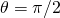and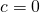meaning the metric reduces to: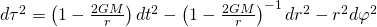or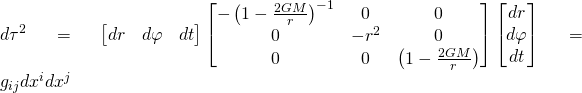where the right hand side uses Einstein notation.

Given coördinatesand first order derivatives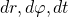at time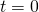, the 2nd order derivatives feeding the simulation can be derived using the geodesic equation: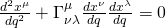whereis derived from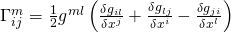again using Einstein notation and withthe inverse matrix of the metric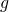.
The exact matricesare provided in the Excel.

An example is provided below and can be simulated in the Excel by setting the starting values toandand the simulation step size to.